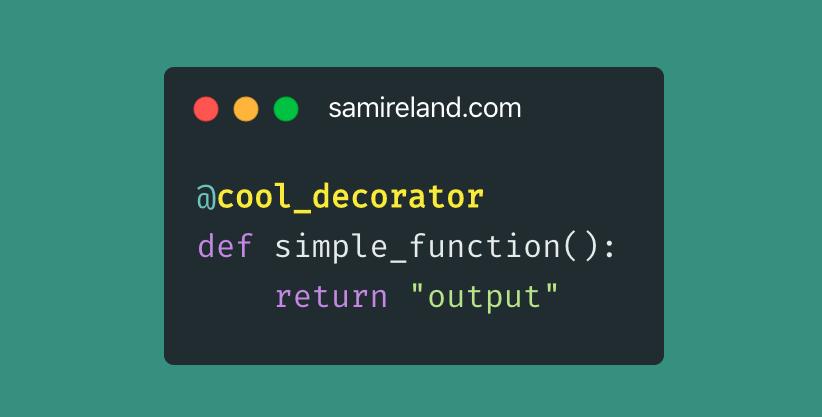# Python 实现一个计时功能的装饰器

hochie

0收藏下面的装饰器clock会打印函数的运行时间

``````# descrbe.py
import time
import functools

def clock(func):
"""this is outer clock function"""

@functools.wraps(func)  # --> 4
def clocked(*args, **kwargs):  # -- 1
"""this is inner clocked function"""
start_time = time.time()
result = func(*args, **kwargs)  # --> 2
time_cost = time.time() - start_time
print(func.__name__ + " func time_cost -> {}".format(time_cost))
return result
return clocked  # --> 3

@functools.lru_cache()  # --> 5
@clock  # --> 6
def fib(n):
"""this is fibonacci function"""
return n if n < 2 else fib(n - 1) + fib(n - 2)

if __name__ == "__main__":
# 如果有 @functools.wraps(func)  # --> 4 大多数情况下我们希望的输出是这样的
fib(1) # 输出 fib func time_cost -> 9.5367431640625e-07
print(fib.__name__)  # 输出 fib
print(fib.__doc__)  # 输出 this is fibonacci function

# 如果没有@functools.wraps(func)  # --> 4
fib(1) # 输出 fib func time_cost -> 9.5367431640625e-07
print(fib.__name__)  # 输出 clocked
print(fib.__doc__)  # 输出 this is inner clocked function``````

• 定义了一个内部函数clocked，它接受任意定位参数以及关键字参数。
• 这行代码可用，是因为clocked的闭包中包含了自由变量func。
• 返回内部的函数，取代被装饰的函数。
• functools.wraps是标准库中拿来即用的装饰器之一,它的作用是协助构建行为良好的装饰器。如果不加functools.wraps(func), 会遮盖被装饰函数的__name__和doc属性，

• functools.lrucache()是非常实用的装饰器，它实现了备忘(memoization)功能。这是一项优化技术，它把耗时的函数的结果保存起来，避免传入相同的参数时重复计算。LRU三个字母是“Least Recently Used”的缩写，表明缓存不会无限制增长，一段时间不用的缓存条目会被扔掉。这样就会显著提高程序的运行效率。lrucache可以使用两个可选的参数来配置。它的方法签名是lru_cache(maxsize=128, typed=False)。maxsize参数指定存储多少个调用的结果。缓存满了之后，旧的结果会被扔掉，腾出空间。为了得到最佳性能，maxsize应该设为2的幂。typed参数如果设为True，把不同参数类型得到的结果分开保存，即把通常认为相等的浮点数和整数参数(如 1 和 1.0)区分开。顺便说一下，因为lrucache使用字典存储结果，而且键根据调用时传入的定位参数和关键字参数创建，所以被lrucache装饰的函数，它的所有参数都必须是可散列的。

• 实际上该处的工作原理如下：

``````@clock  # --> 6
def fib(n):
"""this is fibonacci function"""
return n if n < 2 else fib(n - 1) + fib(n - 2)``````

``fib = clock(fib) ``

fib会作为func参数传给clock。然后，clock函数会返回clocked函数，Python解释器在背后会把 clocked赋值给fib。实际上如果我们没有添加@functools.wraps(func),我们在ipython中导入describe进一步观察：

``````In : import describe
In : describe.fib.__name__
Out: 'clocked'``````

• (1) 记录初始时间 t0。
• (2) 调用原来的 fib 函数，保存结果。
• (3) 计算经过的时间。
• (4) 打印出来格式化收集的数据
• (5) 返回第 2 步保存的结果。
这是装饰器的典型行为:把被装饰的函数替换成新函数，二者接受相同的参数，而且(通常)返回被装饰的函数本该返回的值，同时还会做些额外操作。帖子
视频
声望
粉丝
社区精华内容